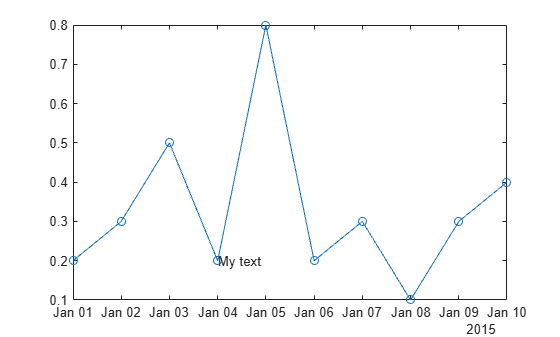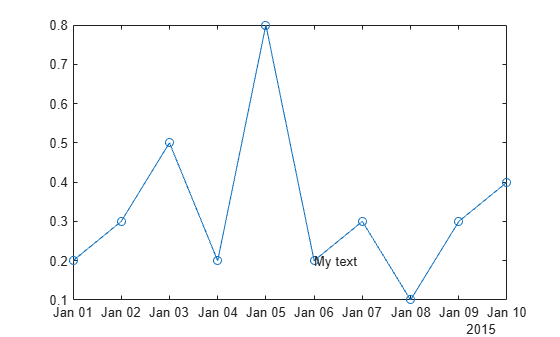ruler2num

Convert data from specific ruler to numeric data

Description

example

num = ruler2num(data,ruler) converts the values in data to numeric values. The ruler input indicates the ruler associated with data, which determines how to map the values to the corresponding numeric values. The output num is the same size as the input data.

Examples

collapse all

Convert a date value to the equivalent numeric value in order to set the Position property of a text object.

Create a plot with dates along the x-axis. Add a text description to the fourth data point and return the text object.

t = datetime(2015,1,1:10);
y = [.2 .3 .5 .2 .8 .2 .3 .1 .3 .4];
plot(t,y,'-o')
txt = text(t(4),y(4),'My text');Change the position of the text to the sixth data point by setting the Position property of the text object. Since the Position property accepts only numeric values, convert the datetime value at the sixth data point to the equivalent numeric value.

ax = gca;
x6 = ruler2num(t(6),ax.XAxis);
txt.Position = [x6 y(6)];Input Arguments

collapse all

Input array, specified as a scalar, vector, or matrix. If data is already numeric, then the output num is equal to the input data.

Ruler associated with the input data, specified as a DatetimeRuler, DurationRuler, NumericRuler, or GeographicRuler object. Specify the ruler object using the XAxis, YAxis, or ZAxis property of the Axes object. For example:

ax = gca;
num = ruler2num(data,ax.XAxis)

If you specify a NumericRuler object and the input data is not numeric, then the output num is determined by:

num = full(double(data))

If you specify a GeographicRuler object, then the output num is equal to the input data.